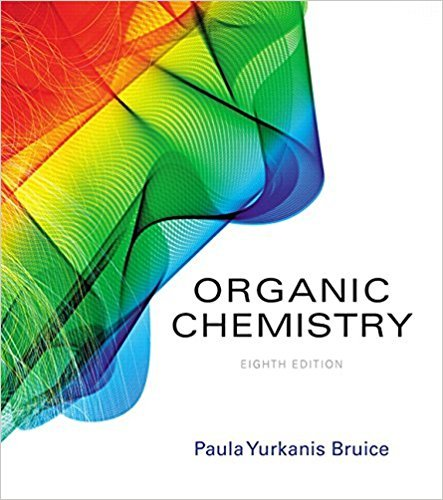×
Get Full Access to Organic Chemistry - 8 Edition - Chapter 5.14 - Problem 44
Get Full Access to Organic Chemistry - 8 Edition - Chapter 5.14 - Problem 44

×

# Solved: a. Draw the condensed structures and give the systematic names for all theISBN: 9780134042282 482

## Solution for problem 44 Chapter 5.14

Organic Chemistry | 8th Edition

• Textbook Solutions
• 2901 Step-by-step solutions solved by professors and subject experts
• Get 24/7 help from StudySoup virtual teaching assistantsOrganic Chemistry | 8th Edition

4 5 1 274 Reviews
28
5
Problem 44

a. Draw the condensed structures and give the systematic names for all the alkenes with molecular formula C6H12, ignoring stereoisomers.(Hint: There are 13.)b. Which of the alkenes have E and Z isomers?c. Which of the alkenes is the most stable?d. Which of the alkenes is the least stable?

Step-by-Step Solution:
Step 1 of 3

CHM 2045 Ch. 3 Notes • Know: mole concept and mole calculations, percent composition, empirical formula & molecular formula determination • Key points about the MOLE concept o The mole maintains the same mass relationships between macroscopic samples as exists between individual chemical entities o The mole relates the number of chemical entities to the mass of a sample of those entities o So we count chemical entities by weighing them 23 o We use Avogadro’s number (6.022 * 10 ) to say that one mole contains 6.022 * 10 Avogadro’s number of ions, molecules, particles, etc. (You must know more than just the number!) o A mole represents a fixed number of chemical entities and has a fixed but different mass for each Mole and Molar Mass Aspirin, (C 9 O8)4 What is aspirin’s molar mass (12.01 g C / mol C, 1.008 g H / mol H, 16.00 g O / mol O) 9 (12.

Step 2 of 3

Step 3 of 3

##### ISBN: 9780134042282

Since the solution to 44 from 5.14 chapter was answered, more than 273 students have viewed the full step-by-step answer. The full step-by-step solution to problem: 44 from chapter: 5.14 was answered by , our top Chemistry solution expert on 03/16/18, 04:59PM. The answer to “a. Draw the condensed structures and give the systematic names for all the alkenes with molecular formula C6H12, ignoring stereoisomers.(Hint: There are 13.)b. Which of the alkenes have E and Z isomers?c. Which of the alkenes is the most stable?d. Which of the alkenes is the least stable?” is broken down into a number of easy to follow steps, and 48 words. Organic Chemistry was written by and is associated to the ISBN: 9780134042282. This full solution covers the following key subjects: . This expansive textbook survival guide covers 127 chapters, and 2116 solutions. This textbook survival guide was created for the textbook: Organic Chemistry, edition: 8.

## Discover and learn what students are asking

Unlock Textbook Solution

Enter your email below to unlock your verified solution to:

Solved: a. Draw the condensed structures and give the systematic names for all the